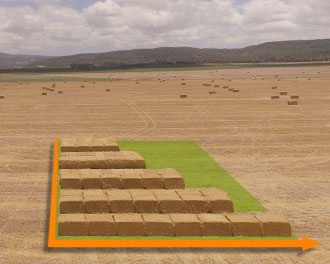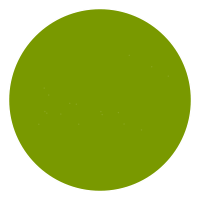## Film for square bales Example 1

There are many varying sizes of square bale wrapped, making calculating the number of bales per roll complex. The quantity of bales wrapped, of any dimension, from one roll of film is dependent on a number of important factors.

• THE BALE SIZE

• THE % STRETCH APPLIED TO THE FILM

• THE NUMBER OF TURNS GIVEN TO THE BALE

By using the following formula, a calculation of the approximate number of square bales, wrapped with 4 or 6 layers of film, from one roll of film can be made

 EXAMPLE – 1.5m x .80m x .85m bale – 4 Layers of film A Add the sum of the bale’s four largest dimensions = 4.7m     (2 x 1.5m + 2 x .85m) B Multiply by 75 (75cm Film width) C Multiply by 0.0025 (25 micron Film thickness) D Multiply by 0.0933 (Film Density) E Divide by 1.7 (Film length plus 70% stretch) F Multiply by 16 (Number of turns of the bale for 4 film layers) = 0.774Kg OF FILM PER BALE G Divide into weight of film on roll (not including core) (approximately 26Kg per 750mm x 1500m roll) 26Kg (total film weight / roll) ÷ 0.774Kg (film weight / bale) = 33 BALES PER ROLL

 EXAMPLE – 1.5m x .80m x .85m – 6 layers of film A Add the sum of the bale’s four largest dimensions = 4.7m     (2 x 1.5m + 2 x .85m) B Multiply by 75 (75cm Film width) C Multiply by 0.0025 (25 micron Film thickness) D Multiply by 0.0933 (Film Density) E Divide by 1.7 (Film length plus 70% stretch) F Multiply by 24 (Number of turns of the bale for 6 film layers) = 1.161Kg OF FILM PER BALE G Divide into weight of film on roll (not including core) (approximately 26Kg per 750mm x 1500m roll) 26Kg (total film weight / roll) ÷ 1.161Kg (film weight / bale) = 22 BALES PER ROLL
Help us improve
To help us improve the quality of our site and give you a better experience, could you take 1 minute to answer a few simple questions!
Did you find what you were looking for today?
What were you looking for?
Please, tell us what you would like to see on the site:
The survey is anonymous
THANK YOU
Help us improve
EXPLORE MORE
Farmer TestimonialsStories from the field See
Quick Contact Get in touch with us
Field SupportCall us for help +353 87 9144244 Twine CalculatorGet your order right CalculateScroll to Top